Function Repository Resource:

# ImportWikipediaTables

Contributed by: César Guerra
 ResourceFunction["ImportWikipediaTables"][url] imports all the available tables from the Wikipedia page located at url. ResourceFunction["ImportWikipediaTables"]["title"] imports the page titled "title". ResourceFunction["ImportWikipediaTables"][…,format] returns the tables in the format specified.

## Details and Options

The format can be any of "ListOfTables" (default), "IconAssociation" or Automatic.
ResourceFunction["ImportWikipediaTables"] takes the following options:
 "AvoidRowsOfUnequalLength" False whether to avoid rows of unequal length "SemanticImportSpecification" {} lists of tables for which to try a SemanticImport "ShowPreview" False whether to show a preview of the imported tables
Tables imported with semantic interpretation are given as Dataset objects. Otherwise tables are nested lists.
ResourceFunction["ImportWikipediaTables"] makes a call to the Import function with "FullData" second argument and then further processes the result to get the tables.

## Examples

### Basic Examples (2)

Import the tables available in the Wikipedia page about "List of countries by forest area". By default the result is a list of tables (nested lists) found in this page:

 In:=Out=See the size of the tables:

 In:=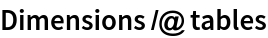Out=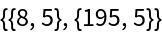Usually, the scraped values are strings, but if the data contains well formatted numbers they are scraped as numeric types. This shows a TextGrid of the first table:

 In:=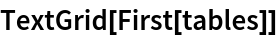Out=If the second argument is specified as "IconAssociation", the result is an Association of table data. The tables are iconized as the "Data" element to shorten long outputs:

 In:=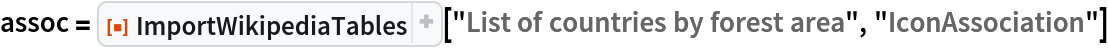Out=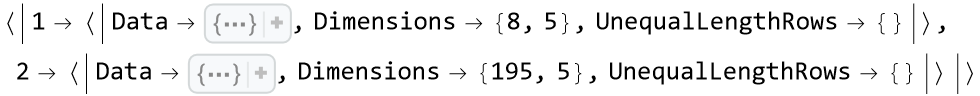Tables can be copy–pasted or extracted programmatically (note that the 1 in the third argument of Query is used to get data from the IconizedObject):

 In:=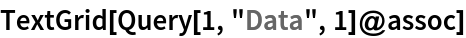Out=### Scope (2)

The key "UnequalLengthRows" contains the indexes of rows which have different lengths from the commonest length of the table. In the following, the first table has the first two rows with a different length from the rest of the table:

 In:=Out=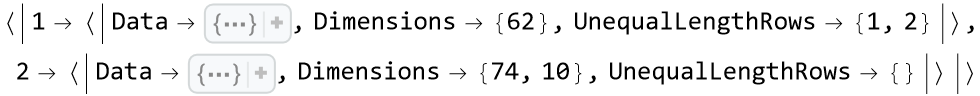This information can be useful for fixing or skipping these rows:

 In:=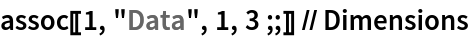Out=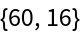In:=Out=### Options (8)

#### AvoidRowsOfUnequalLength (2)

Rows with different length than the commonest length of the table can be automatically skipped:

 In:=Out=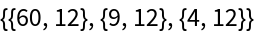Using the default setting shows that the original tables had rows of unequal length:

 In:=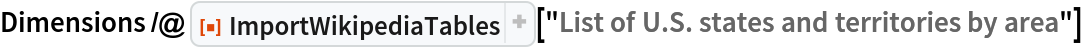Out=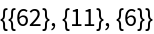#### SemanticImportSpecification (5)

The "SemanticImportSpecification" option can be used to try to semantically import all the tables:

 In:=Out=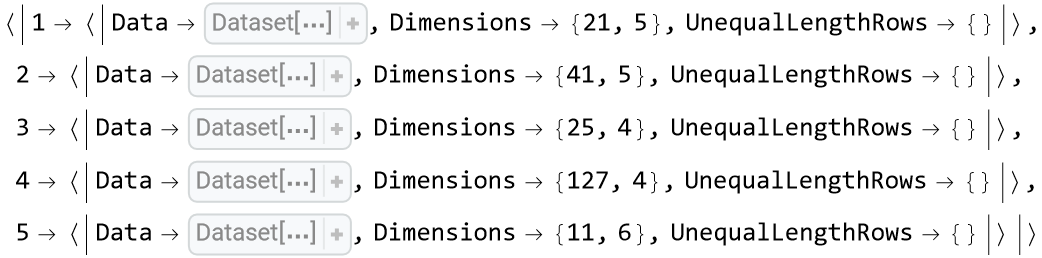Semantically import a specific list of tables:

 In:=Out=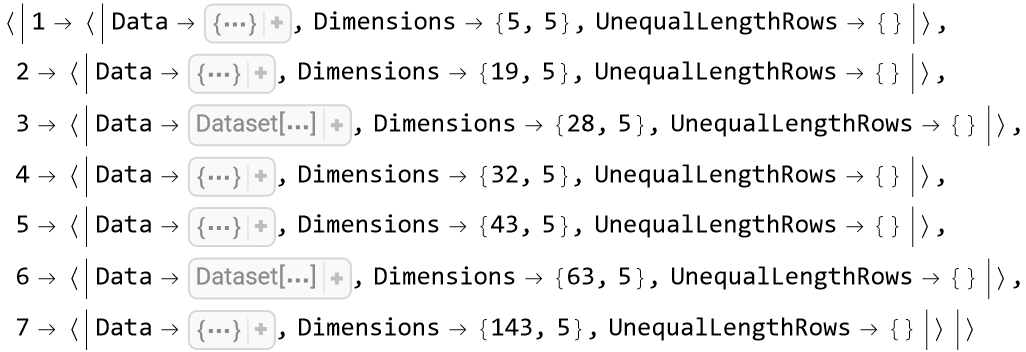The tables can have rows of unequal lengths:

 In:=Out=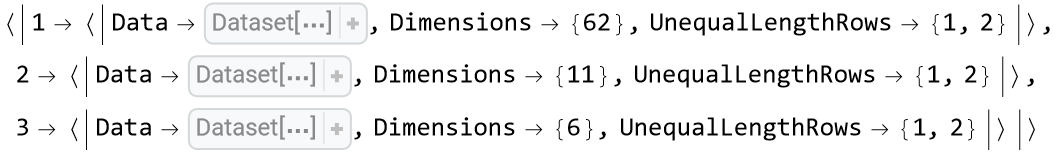An Association of list of types can be passed for the interpretation of each table (see the second argument of SemanticImport):

 In:=Out=Additionally, SemanticImport options can be passed for each table:

 In:=Out=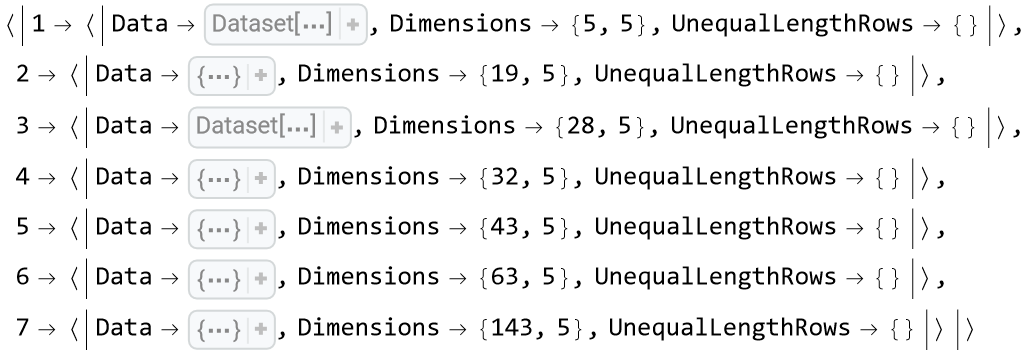#### ShowPreview (1)

Show a preview of each table in a "Print" cell:

 In:=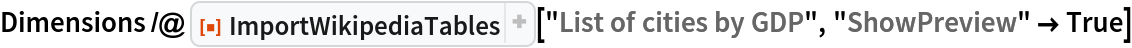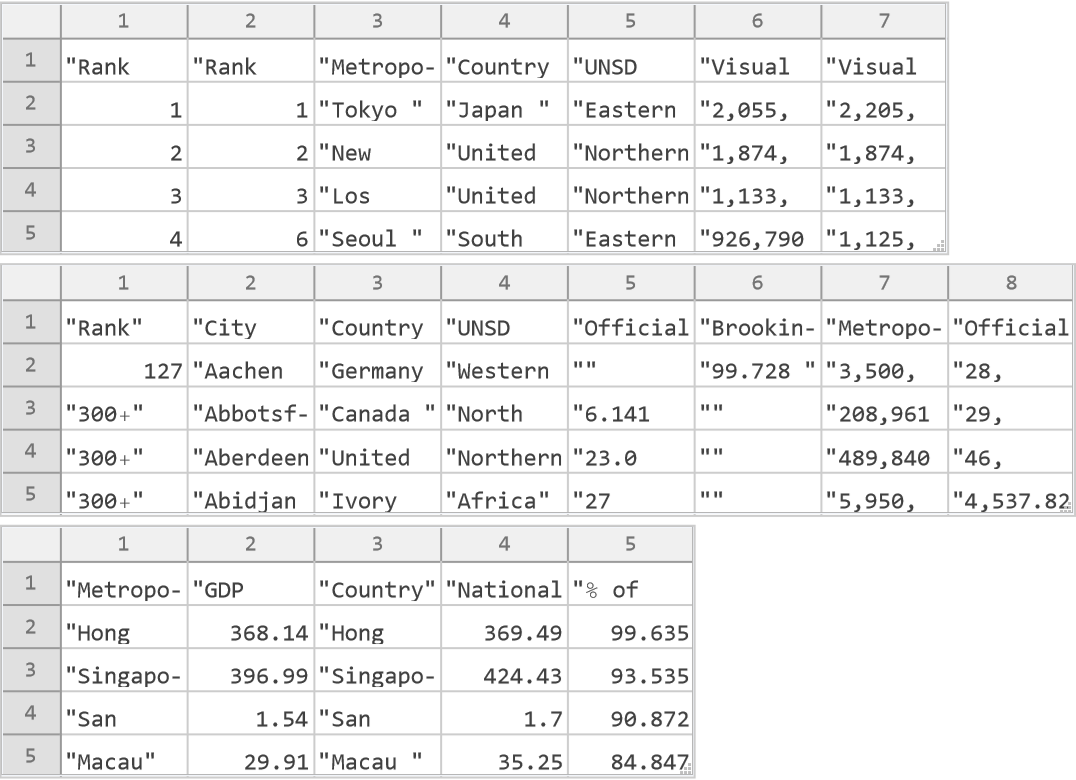Out=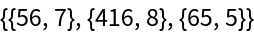### Properties and Relations (2)

Extract the names of the 10 largest lakes by area from a table on Wikipedia:

 In:=Out=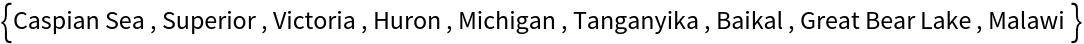In the Wolfram Language, the same data can be gathered directly using the Entity framework and knowledge representation:

 In:=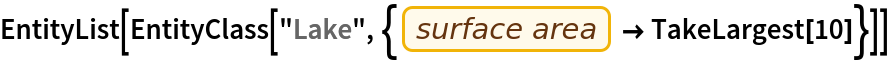Out=Import a table:

 In:=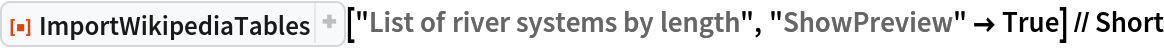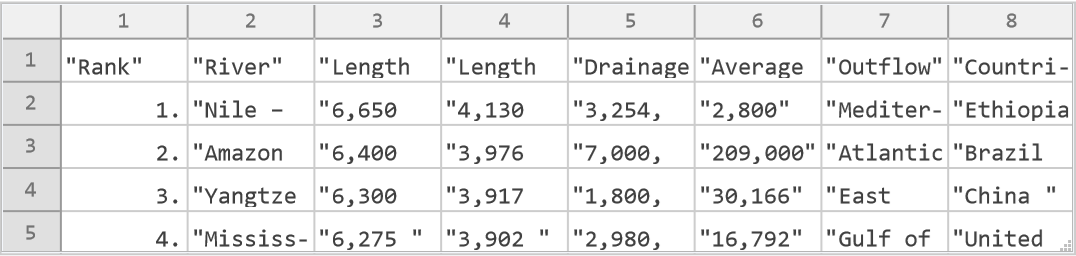Out=For more complex data tables, a direct call to the "Source" element of the Import function can be used, but parsing the result is complicated. For example, here we show the underlying structure of the same table:

 In:=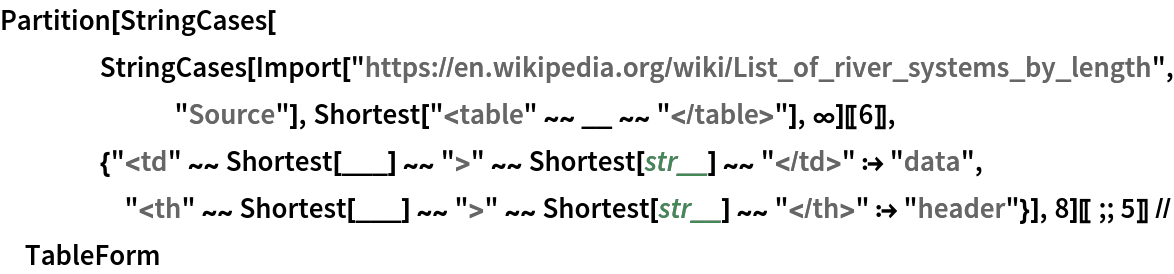Out=### Possible Issues (2)

Unequal length rows can appear at the end of tables, since Wikipedia tables can have headers at the end:

 In:=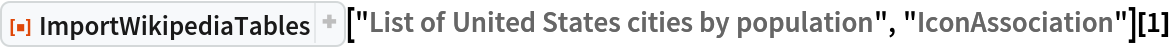Out=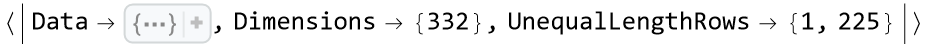Wikipedia tables can also have spanned cells in any place, in those cases they are reported as rows of unequal lengths, so that they can be fixed or deleted:

 In:=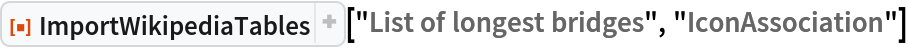Out=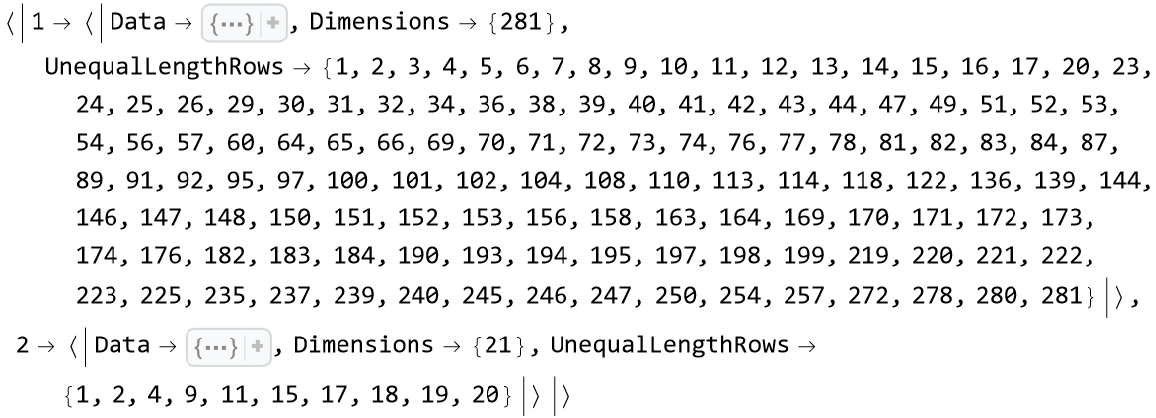In some cases they are due to missing data and can be handled incorrectly by SemanticImport. For example, getting the Cuba GDP from the corresponding Wikipedia table gives an incorrect result, since the values are shifted for this row:

 In:=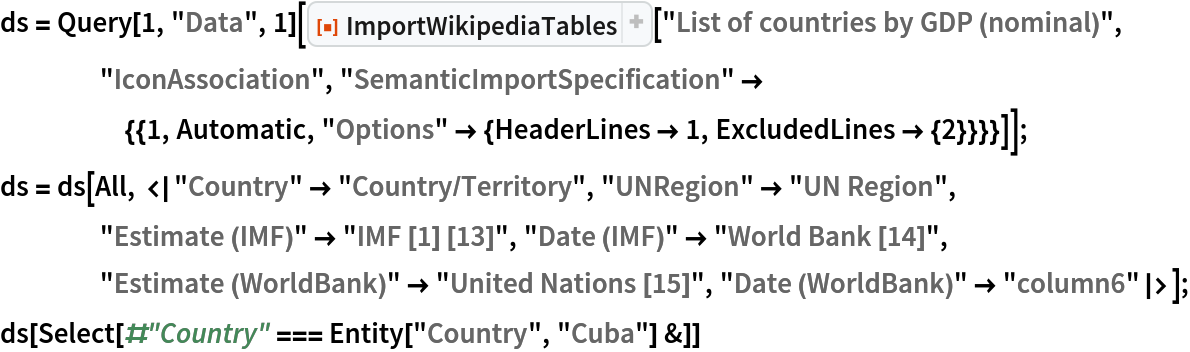Out=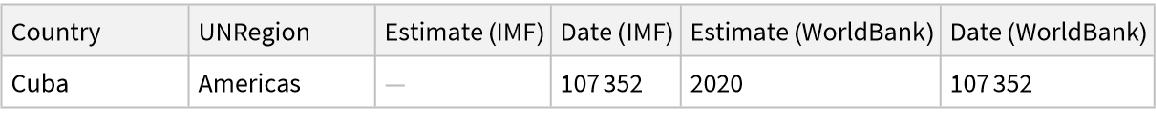In other cases, the unequal length rows can happen because the first column is spanned to group some rows of the table:

 In:=Out=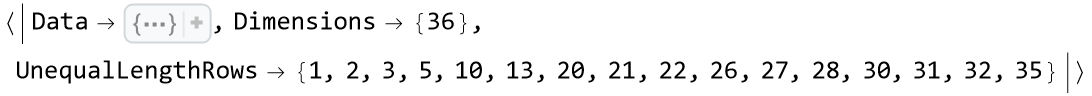To show the spanned strings in the first column we pad the unequal length rows with empty strings:

 In:=Out=### Applications (1)

Histogram of the tallest mountains:

 In:=Out=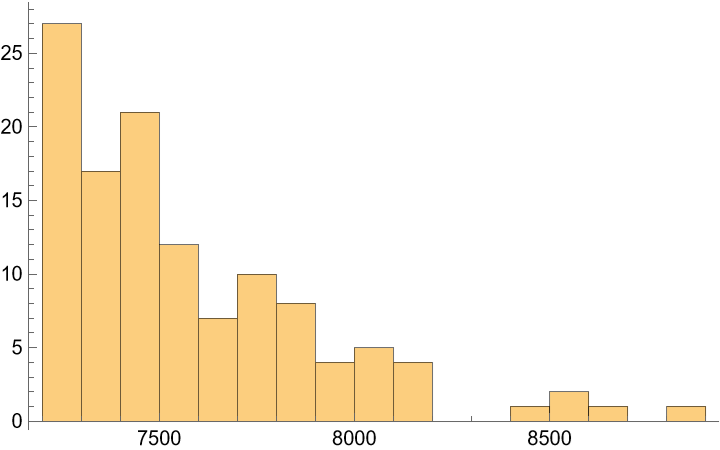Cesar Guerra

## Version History

• 1.0.0 – 15 March 2023